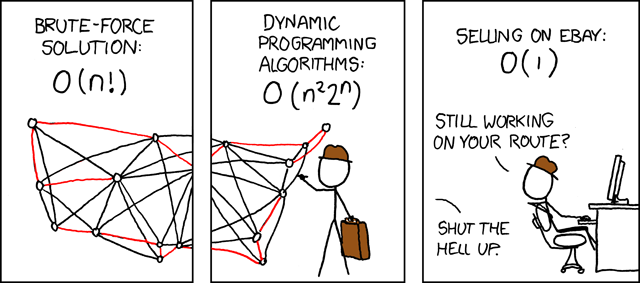# Dynamic Programming

Friday, July 13, 2018

Those who cannot remember the past are condemned to repeat it. — George Santayana

The Fibonacci sequence is defined as follows:

$F_1 = F_2 = 1$ $F_n = F_{n−1} + F_{n−2}$

Let’s write a program to find the nth Fibonacci number.

Notice, some of the numbers are repeatedly evaluated thus this algorithm takes a large amount of running time (exponential). Let’s store the numbers in a dictionary after calculation then we’ll look up the number in the dictionary before evaluation to save time.

The above technique of using a look-up table is called memoization. This process of using a memoization based algorithm is dynamic programming. The running time of dynamic programming is given by

$time = \text{number of subproblems} * time / \text{subproblem} = n * \theta(1) = \theta(n)$

In fact, here we used a top-down approach by solving the subproblems we encountered while solving the problem, there also exists a bottom-up approach of dynamic programming where we first solve all the subproblems before arriving at the problem itself.

A problem must possess the following characteristics in order to be solvable by dynamic programming:

• Optimal substructure: A problem exhibits optimal substructure if an optimal solution to the problem contains within it optimal solutions to subproblems.
• Overlapping subproblems: When the solution of some of the subproblems are same i.e. subproblems share their solutions, they are said to be overlapping. That is, a recursive algorithm for the problem solves the same subproblem repeatedly.

Writes down “1+1+1+1+1+1+1+1 =” on a sheet of paper.
“What’s that equal to?”
Counting “Eight!”
Writes down another “1+” on the left.
“Nine!” “ How’d you know it was nine so fast?”
“So you didn’t need to recount because you remembered there were eight! Dynamic Programming is just a fancy way to say remembering stuff to save time later!” — Quora answer1

Dynamic programming differs from greedy algorithms in one aspect, that is, it first find the optimal solution to subproblems then make the choice while greedy algorithms first make a greedy choice then solve the subproblems.

## Algorithms solvable using Dynamic programming paradigm:

• 0-1 Knapsack problem
• Parenthesization (Matrix chain multiplication)
• Longest Common SubsequenceReferences:
1. Introduction to algorithms / Thomas H. Cormen . . . [et al.].—3rd ed.
2. Dynamic Programmin - Wikipedia
3. How should I explain dynamic programming to a 4-year-old? - Quora